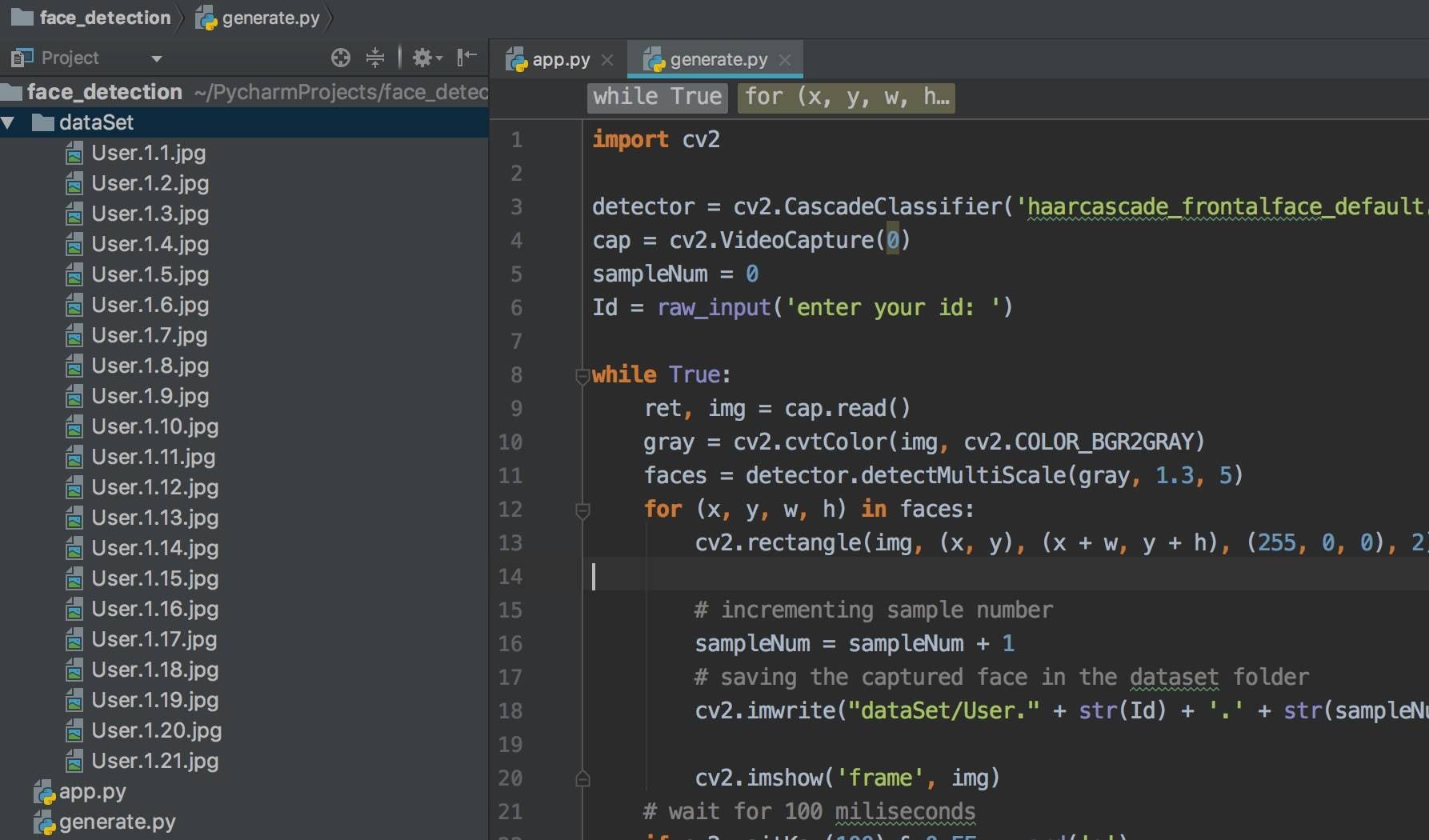# Python-OpenCV人脸识别之数据集生成

1. 创建数据集
2. 训练
3. 识别

## 生成数据集

``````import cv2
cam = cv2.VideoCapture(0)
``````

``````Id = raw_input('enter your id: ')
sampleNum = 0
``````

``````while True:
gray = cv2.cvtColor(img, cv2.COLOR_BGR2GRAY)
faces = detector.detectMultiScale(gray, 1.3, 5)
for (x, y, w, h) in faces:
cv2.rectangle(img, (x, y), (x + w, y + h), (255, 0, 0), 2)

cv2.imshow('frame', img)
if cv2.waitKey(1) & 0xFF == ord('q'):
break
``````

``````while True:
gray = cv2.cvtColor(img, cv2.COLOR_BGR2GRAY)
faces = detector.detectMultiScale(gray, 1.3, 5)
for (x, y, w, h) in faces:
cv2.rectangle(img, (x, y), (x + w, y + h), (255, 0, 0), 2)

# 增加例子数
sampleNum = sampleNum + 1
# 把照片保存到数据集文件夹
cv2.imwrite("dataSet/user." + str(Id) + '.' + str(sampleNum) + ".jpg", gray[y:y + h, x:x + w])

cv2.imshow('frame', img)
if cv2.waitKey(1) & 0xFF == ord('q'):
break
``````

``````while True:
gray = cv2.cvtColor(img, cv2.COLOR_BGR2GRAY)
faces = detector.detectMultiScale(gray, 1.3, 5)
for (x, y, w, h) in faces:
cv2.rectangle(img, (x, y), (x + w, y + h), (255, 0, 0), 2)

# 增加例子数
sampleNum = sampleNum + 1
# 把照片保存到数据集文件夹
cv2.imwrite("dataSet/User." + str(Id) + '.' + str(sampleNum) + ".jpg", gray[y:y + h, x:x + w])  #

cv2.imshow('frame', img)
# 延迟100毫秒
if cv2.waitKey(100) & 0xFF == ord('q'):
break
# 超过20张就可以停了
elif sampleNum > 20:
break
``````

``````cap.release()
cv2.destroyAllWindows()
``````

``````import cv2

cap = cv2.VideoCapture(0)
sampleNum = 0
Id = raw_input('enter your id: ')

while True:
gray = cv2.cvtColor(img, cv2.COLOR_BGR2GRAY)
faces = detector.detectMultiScale(gray, 1.3, 5)
for (x, y, w, h) in faces:
cv2.rectangle(img, (x, y), (x + w, y + h), (255, 0, 0), 2)

# incrementing sample number
sampleNum = sampleNum + 1
# saving the captured face in the dataset folder
cv2.imwrite("dataSet/User." + str(Id) + '.' + str(sampleNum) + ".jpg", gray[y:y + h, x:x + w])  #

cv2.imshow('frame', img)
# wait for 100 miliseconds
if cv2.waitKey(100) & 0xFF == ord('q'):
break
# break if the sample number is morethan 20
elif sampleNum > 20:
break

cap.release()
cv2.destroyAllWindows()
``````

## 生成结果